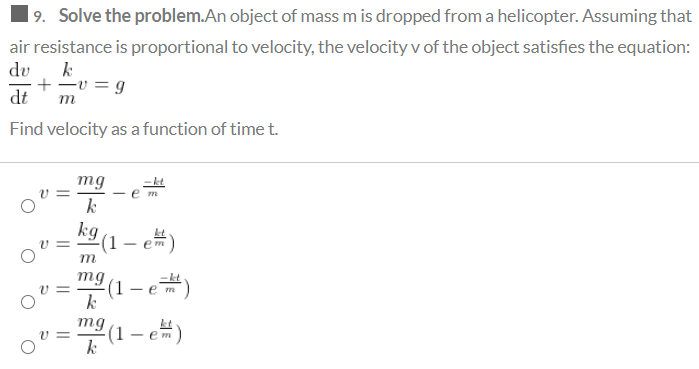# Question Solved1 Answer19. Solve the problem.An object of mass m is dropped from a helicopter. Assuming that air resistance is proportional to velocity, the velocity v of the object satisfies the equation: du k + -0 = 9 dt Find velocity as a function of time t. m mg k em - em V = m mg (1) k kg (1-e e) mg (1 - e) U = kTranscribed Image Text: 19. Solve the problem.An object of mass m is dropped from a helicopter. Assuming that air resistance is proportional to velocity, the velocity v of the object satisfies the equation: du k + -0 = 9 dt Find velocity as a function of time t. m mg k em - em V = m mg (1) k kg (1-e e) mg (1 - e) U = k
More
Transcribed Image Text: 19. Solve the problem.An object of mass m is dropped from a helicopter. Assuming that air resistance is proportional to velocity, the velocity v of the object satisfies the equation: du k + -0 = 9 dt Find velocity as a function of time t. m mg k em - em V = m mg (1) k kg (1-e e) mg (1 - e) U = k